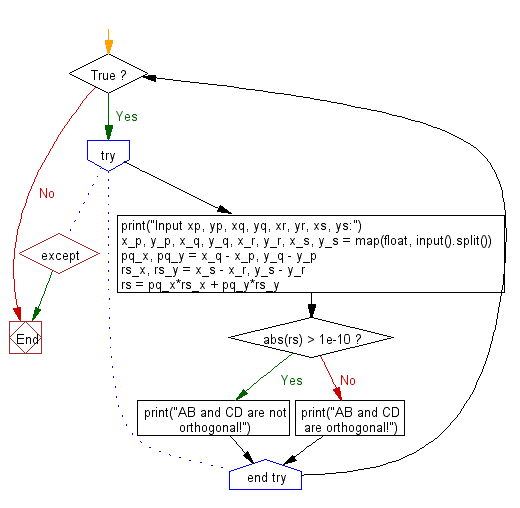﻿ Python: Test AB and CD are orthogonal or not - w3resource# Python: Test AB and CD are orthogonal or not

## Python Basic - 1: Exercise-55 with Solution

There are four different points on a plane, P(xp,yp), Q(xq, yq), R(xr, yr) and S(xs, ys). Write a Python program to test AB and CD are orthogonal or not.

Input:
xp,yp, xq, yq, xr, yr, xs and ys are -100 to 100 respectively and each value can be up to 5 digits after the decimal point It is given as a real number including the number of.
Output:
Output AB and CD are not orthogonal! or AB and CD are orthogonal!.

Sample Solution:

Python Code:

``````while True:
try:
print("Input xp, yp, xq, yq, xr, yr, xs, ys:")
x_p, y_p, x_q, y_q, x_r, y_r, x_s, y_s = map(float, input().split())
pq_x, pq_y = x_q - x_p, y_q - y_p
rs_x, rs_y = x_s - x_r, y_s - y_r
rs = pq_x*rs_x + pq_y*rs_y
if abs(rs) > 1e-10:
print("AB and CD are not orthogonal!")
else:
print("AB and CD are orthogonal!")
except:
break
``````

Sample Output:

```Input xp, yp, xq, yq, xr, yr, xs, ys:
4.5 -2.5 -2.5 4.5 3.5 3.5 3.8 -3.5
AB and CD are not orthogonal!
```

Flowchart:Python Code Editor:

Have another way to solve this solution? Contribute your code (and comments) through Disqus.

What is the difficulty level of this exercise?

Test your Programming skills with w3resource's quiz.

﻿

## Python: Tips of the Day

How to make a flat list out of list of lists?

Given a list of lists l

```flat_list = [item for sublist in l for item in sublist]
```

which means:

```flat_list = []
for sublist in l:
for item in sublist:
flat_list.append(item)
```

is faster than the shortcuts posted so far. (l is the list to flatten.) Here is the corresponding function:

flatten = lambda l: [item for sublist in l for item in sublist]

As evidence, you can use the timeit module in the standard library:

```\$ python -mtimeit -s'l=[[1,2,3],[4,5,6], , [8,9]]*99' '[item for sublist in l for item in sublist]'
10000 loops, best of 3: 143 usec per loop
\$ python -mtimeit -s'l=[[1,2,3],[4,5,6], , [8,9]]*99' 'sum(l, [])'
1000 loops, best of 3: 969 usec per loop
\$ python -mtimeit -s'l=[[1,2,3],[4,5,6], , [8,9]]*99' 'reduce(lambda x,y: x+y,l)'
1000 loops, best of 3: 1.1 msec per loop
```

Explanation: the shortcuts based on + (including the implied use in sum) are, of necessity, O(L**2) when there are L sublists -- as the intermediate result list keeps getting longer, at each step a new intermediate result list object gets allocated, and all the items in the previous intermediate result must be copied over (as well as a few new ones added at the end). So, for simplicity and without actual loss of generality, say you have L sublists of I items each: the first I items are copied back and forth L-1 times, the second I items L-2 times, and so on; total number of copies is I times the sum of x for x from 1 to L excluded, i.e., I * (L**2)/2.

The list comprehension just generates one list, once, and copies each item over (from its original place of residence to the result list) also exactly once.

Ref: https://bit.ly/3dKsNTR